# Statics problem: A ramp is supported by two cables

• Engineering
Homework Statement:
The ramp ABCD is supported by cables at corners C and D. The tensile force in both cables is 420N. Determine the moment about point A produced by the force exerted at point C.
Relevant Equations:
Moment at A (MA) = rGA x TCG.
Where rGA = GA/|GA|
TCG = 420N
Problem illustration: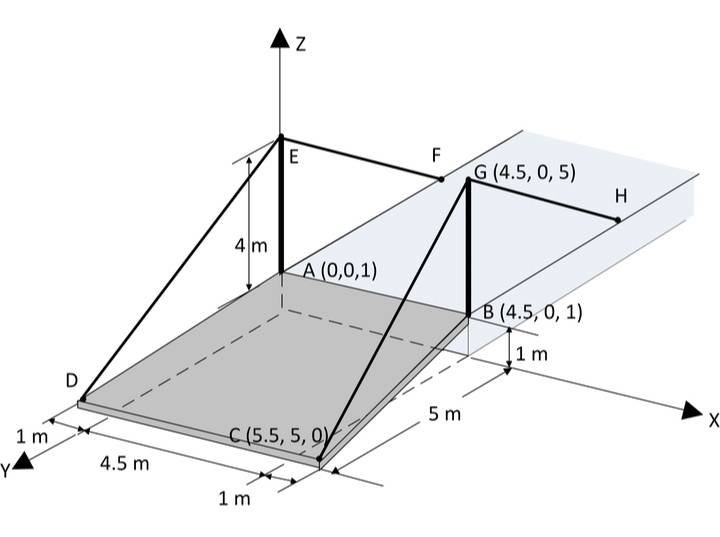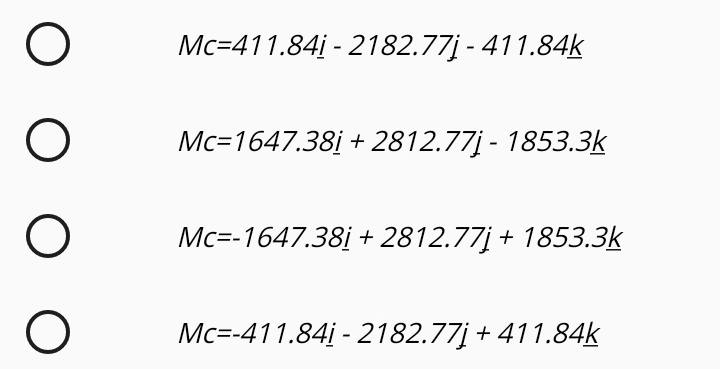I don't understand why it says Mc if it is asking for the moment at A, not C. But maybe I am getting something wrong.

So with the formulas I posted above, I have this: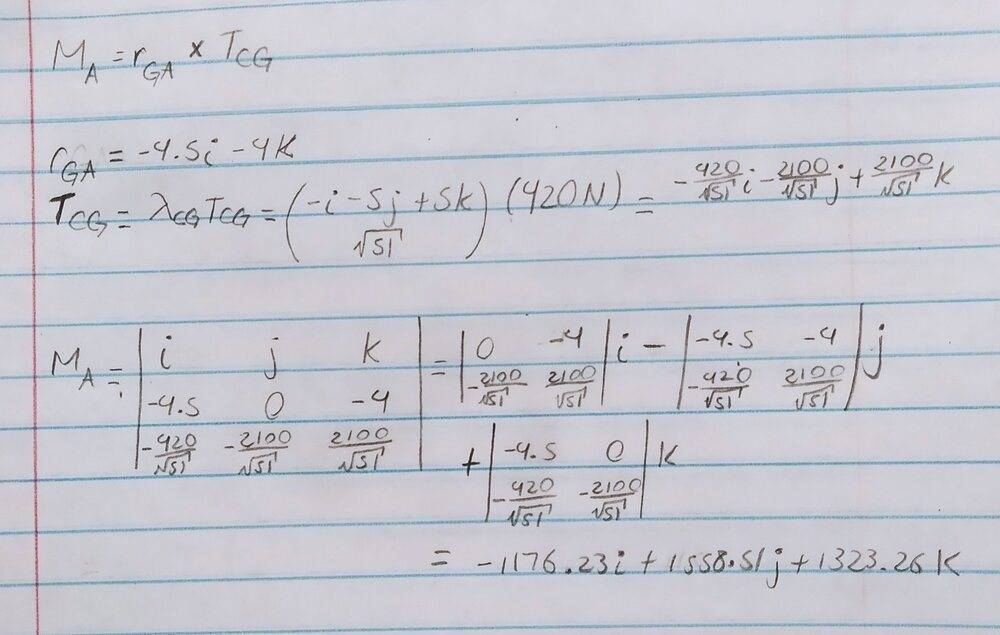Last edited by a moderator:

Delta2
Homework Helper
Gold Member
Your mistake is that you insert ##r_{GA}## in the formula for the moment while you should insert ##r_{CA}##. It is the moment about point A, of the force that is applied at point C not at point G.

What you did is calculate the moment about A, of the force that is applied at point G which is not what is asked for. The forces at G and C might be equal in magnitude , but the point of application differs (and the direction reverses).

Your mistake is that you insert ##r_{GA}## in the formula for the moment while you should insert ##r_{CA}##. It is the moment about point A, of the force that is applied at point C not at point G.

What you did is calculate the moment about A, of the force that is applied at point G which is not what is asked for. The forces at G and C might be equal in magnitude , but the point of application differs (and the direction reverses).

I understand what you mean. In fact the approach you mentioned is the one I thought first. I did it and the answer I got wasn't included on all the possible answers.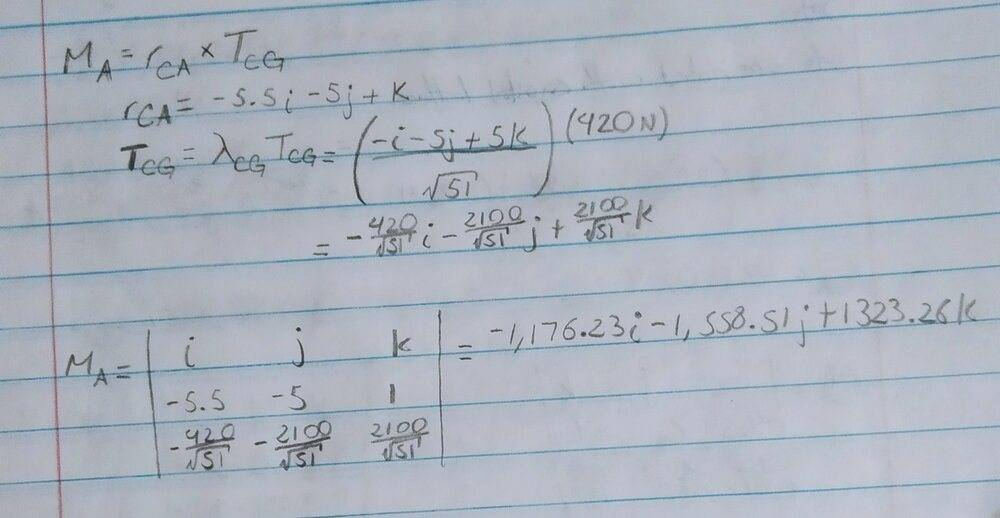The other approach I posted above, with the ##r_{GA}## was based on an example I found, asking the same thing of the same structure, but with different distance units. Why does ##r_{GA}## is used, instead of ##r_{CA}## ?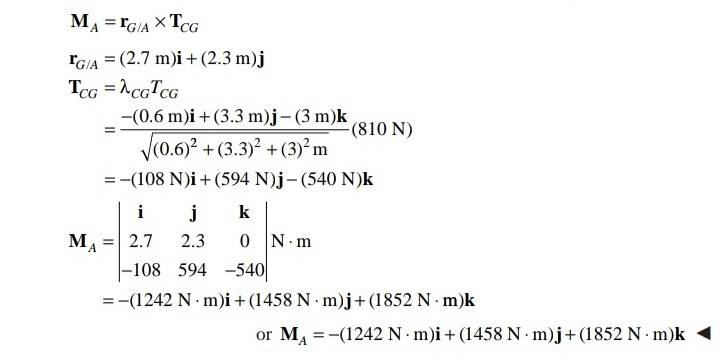Update: As I suspected and mentioned before, the possible answers are wrong because they are referring to the moment ##M_C## and not ##M_A## .

#### Attachments

Last edited:
Delta2
Homework Helper
Gold Member
Well sorry , I now see that it is the same thing whether you take the arm to be ##r_{CA}## or ##r_{GA}## because it is ##r_{CA}=r_{GA}+r_{CG}## and when we take the cross product with ##T_{CG}## the term ##r_{CG}\times T_{CG}=0## vanishes. You indeed calculated both to be equal which verifies it.

I don't know why the answer doesn't match one of the options. It might be because they are referring to the moment ##M_C## as you say, i.e moment about point C.

•Camailee